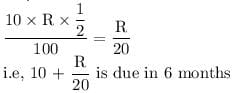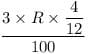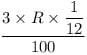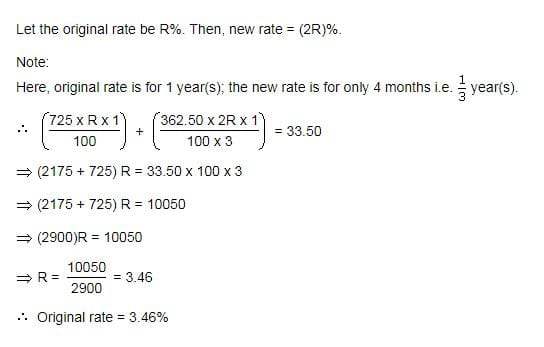# Test: Simple Interest- 2

## 10 Questions MCQ Test CSAT Preparation for UPSC CSE | Test: Simple Interest- 2

Description
Attempt Test: Simple Interest- 2 | 10 questions in 10 minutes | Mock test for Banking Exams preparation | Free important questions MCQ to study CSAT Preparation for UPSC CSE for Banking Exams Exam | Download free PDF with solutions
QUESTION: 1

### If the simple interest on a certain sum of money after 3 1/8 years is 1/4 of the principal, what is the rate of interest per annum?

Solution:

Take P=x
given T=25/8
& I=1/4x
w.k.t I=PTR/100
1/4x =x*25/8*R/100
R = 8%

QUESTION: 2

### What annual payment will discharge a debt of Rs. 6450 due in 4 years at 5% per annum?

Solution:

4x + (3x/20 + 2x/20 + x/20) = 6450
4x + 6x/20 = 6450
43x = 64500
x = 1500

QUESTION: 3

### A sum of Rs. 10 is given as a loan to be returned in 6 monthly installments at Rs.3. What is the rate of interest?

Solution:

Amount borrowed = Rs.10
Let rate of interest = R%
Simple Interest for Rs.10 for 6 months at R% =Payment after 1st month = Rs.3
Interest for this Rs.3 for the remaining 4 months =Payment after 5th month = Rs.3
Interest for this Rs.3 for the remaining 1 month   =Payment after 6th month = Rs.3 and this closes the loan

Hence the rate of interest is 640%

QUESTION: 4

A sum of Rs. 725 is lent in the beginning of a year at a certain rate of interest. After 8 months, a sum of Rs. 362.50 more is lent but at the rate twice the former. At the end of the year, Rs. 33.50 is earned as interest from both the loans. What was the original rate of interest?

Solution:QUESTION: 5

A lent Rs. 5000 to B for 2 years and Rs. 3000 to C for 4 years on simple interest at the same rate of interest and received Rs. 2200 in all from both of them as interest. The rate of interest per annum is:

Solution:
QUESTION: 6

An automobile financier claims to be lending money at simple interest, but he includes the interest every six months for calculating the principal. If he is charging an interest of 10%, the effective rate of interest becomes:

Solution:

B is the correct option. Solution
6 months interest will be 5%
next 6 months interest will be 5%
percentage effect = 5 + 5 +25/100
= 10.25 Ans

QUESTION: 7

If a sum of Rs. 9 is lent to be paid back in 10 equal monthly installments of re. 1 each, then the rate of interest is

Solution:

The correct answer is D as
Loan amount =Rs. 9=Rs. 9
Let rate of interest be R%
Simple Interest on Rs. 9 Rs. 9 for 1010 months
=(9×R×10/12)/100=3R/40
Therefore, amount due in 1010 months =9+3R/40=9+3R/40
Payment after first month =1=1
Interest on 11 for remaining nine months =1×R×912×100=1×R×9/12×100
Payment after second month =1=1
Interest on 11 for remaining eight months =1×R×812×100=1×R×8/12×100
⋯⋯
Payment after ninth month =1=1
Interest on 11 for the remaining one month =1×R×112×100=1×R×112×100
Payment after tenth month =1=1 and this will close the loan.
Therefore,
1×10+R(9+8+⋯+1)/12×100=9+3/R40⇒1+3R/80=3R/40⇒3R/80=1⇒R=26.67

QUESTION: 8

Divide Rs. 2379 into 3 parts so that their amount after 2,3 and 4 years respectively may be equal, the rate of interest being 5% per annum at simple interest. The first part is

Solution:

The correct answer is D  as
let assume 3 parts of amount are x,y,z
x+y+z=2379
SI for x= x*5*2/100⇒ x/10
Total amount after 2 years - x+(x/10)= 11x/10 ------------(1)
SI for y= y*5*3/100=> 15y/100
Total amount after 3 years - y+(15y/100) = 115y/100 -------(2)
SI for z= z*5*4/100=> z/5
Total amount after 4 years - z+(z/5) = 6z/5 ---------------(3)

As per the conditions "3 parts amounts after 2, 3 and 4 years respectively may be equal" (1) = (2) = (3)
based on the above condition and x+y+z=2379, we can get x= 828, y= 792 and z= 759

QUESTION: 9

A lends Rs. 1500 to B and a certain sum to C at the same time at 8% per annum simple interest. If after 4 years, A altogether receives Rs. 1400 as interest from B and C, then the sum lent to C is A.

Solution:

A is the correct option.
Simple interest on the total money lent at
8% FOR 4 YEARS = 1400
Total money lent
= 100/8 × 1400/4 =4375
Therefore, money lent to C
= 4375- 1400 = 2875

QUESTION: 10

A sum of Rs. 14,000 amounts to Rs. 22,400 in 12 years at the rate of simple interest. What is the rate of interest?

Solution:

Amount = 22400, P = 14000, T = 12yrs
SI = 22400 - 14000
= 8400
Rate = (8400*100)/(12*14000)
= 5%Use Code STAYHOME200 and get INR 200 additional OFF Use Coupon Code